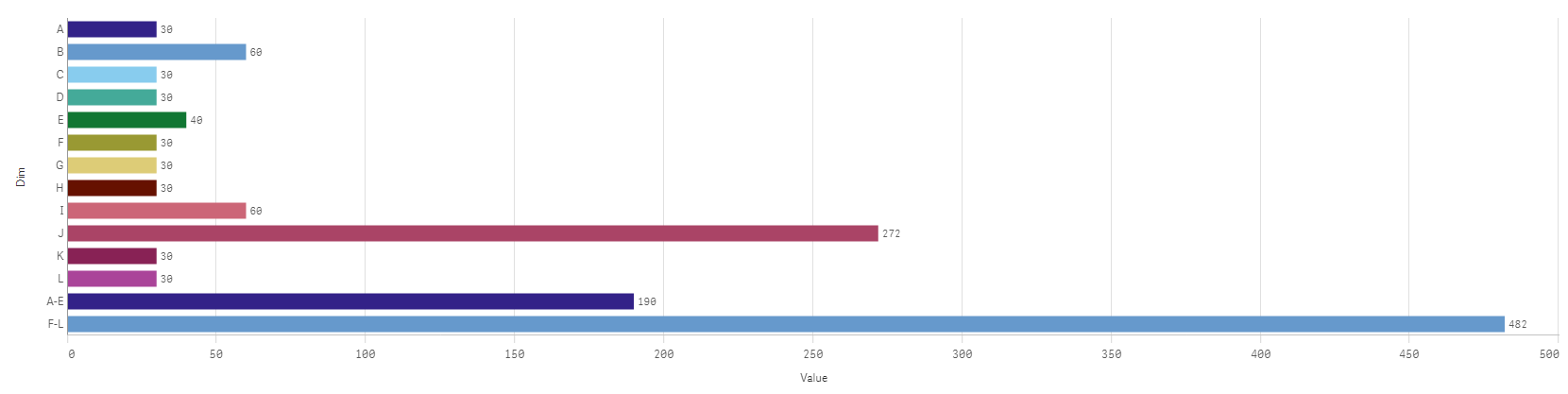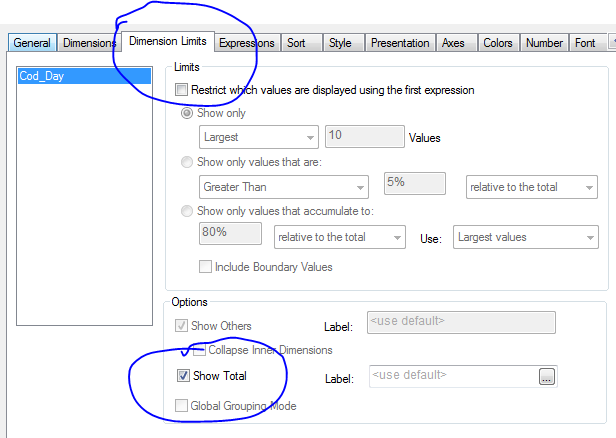# Qlik Sense App DevelopmentNot applicable

## How to add the sum of dimensions in a bar graph

So I have a visualization where I am listing the savings of different categories. For example, I have categories A-E. How do I go about adding the total savings of Categories A-E? Preferably it would be displayed to the right of the other categories divided by a bar if possible.

Tags (3)
1 Solution

Accepted Solutions
Honored Contributor II

## Re: How to add the sum of dimensions in a bar graph

Hi Toufique,

Are you trying to achieve this in a bar chart? Sub totals are not available in Sense yet but you have work around and can be done using the script.

In the image below, the aggregated values A-E and F-L are displayed to the bottom of the chart as you needed.data:

Dim,Value

A,30

B,60

C,30

D,30

E,40

F,30

G,30

H,30

I,60

J,272

K,30

L,30

];

Mapp:

Dim,Cat

A,A-E

B,A-E

C,A-E

D,A-E

E,A-E

F,F-L

G,F-L

H,F-L

I,F-L

J,F-L

K,F-L

L,F-L

];

xData:

ApplyMap('Mapp', Dim) as Cat

Resident data;

Concatenate

yData:

load rowno() as Counter,Cat as Dim, Sum(Value) as Value

Resident xData group by Cat;

drop table data;

Sort the visualization by Counter

Thanks and Regards,

Sangram Reddy.

9 RepliesMVP

## Re: How to add the sum of dimensions in a bar graph

Use 'Show Total' on the Dimension Limits tab:Honored Contributor II

## Re: How to add the sum of dimensions in a bar graph

Hi Toufique,

As Sunny T mentioned, there is a show totals option in QlikSense table as well.Not applicable

## Re: How to add the sum of dimensions in a bar graph

This is embarrassing but I do not know how you got to that window?

I am using Qlik Sense Desktop is that makes a difference, but I have not seen a single window in that format.MVP

## Re: How to add the sum of dimensions in a bar graph

No need to be embarrassed. It was my mistake assuming that you are using QlikView. Sangram Reddy‌ should be able to point you out where to go in order to get total.

Best,

SunnyNot applicable

## Re: How to add the sum of dimensions in a bar graphNot applicable

## Re: How to add the sum of dimensions in a bar graph

Hello Sangram,

So I am using Qlik Sense Desktop, and thus cannot use the solution Sunny provided. Can you help me complete this action in Qlik Sense Desktop?

Honored Contributor II

## Re: How to add the sum of dimensions in a bar graph

Hi Toufique,

Are you trying to achieve this in a bar chart? Sub totals are not available in Sense yet but you have work around and can be done using the script.

In the image below, the aggregated values A-E and F-L are displayed to the bottom of the chart as you needed.data:

Dim,Value

A,30

B,60

C,30

D,30

E,40

F,30

G,30

H,30

I,60

J,272

K,30

L,30

];

Mapp:

Dim,Cat

A,A-E

B,A-E

C,A-E

D,A-E

E,A-E

F,F-L

G,F-L

H,F-L

I,F-L

J,F-L

K,F-L

L,F-L

];

xData:

ApplyMap('Mapp', Dim) as Cat

Resident data;

Concatenate

yData:

load rowno() as Counter,Cat as Dim, Sum(Value) as Value

Resident xData group by Cat;

drop table data;

Sort the visualization by Counter

Thanks and Regards,

Sangram Reddy.Not applicable

## Re: How to add the sum of dimensions in a bar graph

Does this mean I need to manually enter in the value for each category to get the total?

Honored Contributor II

## Re: How to add the sum of dimensions in a bar graph

Not at all, you must be having a table which specify the category s isn't it? So that can be used for mapping purpose.